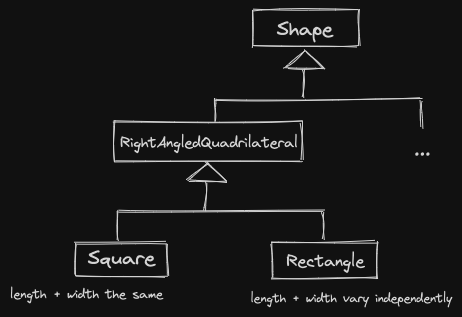SOLID Design Principles

# Liskov Substitution Principle (LSP) §

The Liskov Substitution Principle states that objects of a superclass should be replaceable with objects of its subclasses without breaking the application.

LSP enforces something discussed so far: public inheritance should model an is-a relationship.

If class B inherits from class A: we can use pointers / references to B objects in the place of pointers / references to A objects: C++ gives us this.

Liskov Substitution is stronger: not only can we perform the substitution, but we should be able to do so at any place in the program, without affecting its correctness.

So precisely:

• If an invariance is true for class A, then it should also be true for class B
• If an invariant is true for method A::f, and B overrides f, then this invariant must be true for B::f
• If B::f overrides A::f
• If A::f has a precondition P and a postcondition Q, then B::f should have a precondition P' such that P=>P' and a postcondition Q' such that Q'=>Q
• B::f needs a weaker precondition and a stronger postcondition

Ex: Contravariance problem

• Happens whenever we have a binary operator where the other parameter is the same type as *this.
class Shape {
public:
virtual bool operator==(const Shape& other) const;
}
class Circle: public Shape {
public:
bool operator==(const Shape& other) const override;

}

As we’ve seen before, we must take in the same parameter when overriding. C++ enforces this, which actually enforces LSP for us.

1. A Circle is-a Shape
2. A Shape can be compared with other Shapes
3. A Circle can be compared with any other Shape

To satisfy LSP, we must support comparison between different types of Shapes.

bool Circle::operator==(const Shape& other) const {
if (typeid(other) != typeid(Circle)) return false;
const Circle& cother=static_cast<const Circle&>(other);
...
}

 typeidreturnsstd::type_info objects that can be compared.

dynamic_cast: Is other a Circle, or a subclass of Circle? typeid: Is other exactly a Circle?

typeid uses the dynamic-type so long as the class has at least one virtual method.

### Example of Violation of LSP §

Ex: Is a Square a Rectangle? 4th Grader: Yes, a Square is a rectangle.

A square has all the properties of a rectangle.

class Rectangle {
int length, width;
public:
Rectangle(int l, int w): length{l}, width{w} {}
int getLength() const;
int getWidth() const;
virtual void setWidth(int w) {width=w;};
virtual void setLength(int l) {length=l;};
int area() const {return length * width;};
};

class Square: public Rectangle {
public:
Square(int s): Rectangle{s,s} {}
void setWidth(int w) override {
Rectangle::setWidth(w);
Rectangle::setLength(w);
}
void setLength(int l) override {
Rectangle::setWidth(l);
Rectangle::setLength(l);
}
};

int f(Rectangle& r) {
r.setLength(10);
r.setWidth(20);
return r.area(); // surely this returns 200
}

// But:
Square s{100};
f(s); // gives us 400

What is the issue here?

we expect postcondition for Rectangle::setWith to be that the width is set and nothing else changes. But this is violated by our Square` class.

Violates LSP, does not satisfy an is-a relationship. Conclusion: Square are not Rectangles.

Possibility: Restructure inheritance hierarchy to make sure LSP is respected.In general: how can we prevent LSP violations?

We should restrain what our subclasses can do, only allows them to customize what is truly necessary.

We can use a design pattern: Template Method Pattern, to make this process easier.